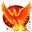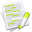#wx.lib.delayedresult.Struct¶

An object that has attributes built from the dictionary given in constructor. So ss=Struct(a=1, b=’b’) will satisfy assert ss.a == 1 and assert ss.b == ‘b’.

##Class Hierarchy¶Inheritance diagram for class Struct:

##Methods Summary¶

 __init__ Initialize self. See help(type(self)) for accurate signature.

##Class API¶

class Struct

An object that has attributes built from the dictionary given in constructor. So ss=Struct(a=1, b=’b’) will satisfy assert ss.a == 1 and assert ss.b == ‘b’.

### Methods¶

__init__(self, **kwargs)

Initialize self. See help(type(self)) for accurate signature.